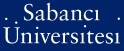# Ramification in some non-galois extension of function fields

Polat, Özgür (2009) Ramification in some non-galois extension of function fields. [Thesis]Preview
PDF - Requires a PDF viewer such as GSview, Xpdf or Adobe Acrobat Reader
146Kb

## Abstract

Throughout this thesis, we denote by k an algebraically closed field of characteristic p > 0, and K /k is a function field over k. We consider extensions L = K(r); where r is a root of one of the following, f(x) = x^p + bx + d (1) f(x) = x^p + bx^(p-1)+ d (2) with b; d in K different from zero. For each polynomial listed above, we will describe ramification behavior of places P of K in the extension L=K, i.e. we will determine ramification index and different exponent of the places P' of L lying above P.

Item Type: Thesis Yükseköğretim Kurulu Tez Merkezi Tez No: 418653. Function fields. -- Galois group. -- Ramification index. -- Different exponent. Q Science > QA Mathematics 34848 IC-Cataloging 24 May 2018 12:10 25 Mar 2019 17:28

Repository Staff Only: item control page